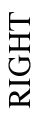### Home > CCG > Chapter 9 > Lesson 9.1.4 > Problem9-49

9-49.

The mat plan for a three-dimensional solid is shown at right.

1. On your paper, draw the front, right, and top views of this solid.

Find the largest number in each column.

For each column, model that number of unit cubes stacked vertically.

Find the right view using the same technique, except going by row instead of column.

Find the top view by noting which squares have at least one unit cube.

2. Find the volume and surface area of the solid.

The volume can be found by finding the sum of all the numbers on the mat plan.

 $3$ $2$ $0$$1$ $4$ $1$ $0$ $3$ $2$ FRONTMat Plan

Use the eTool below to model the solid.
Click the link at right for the full version of the eTool: 9-49 HW eTool